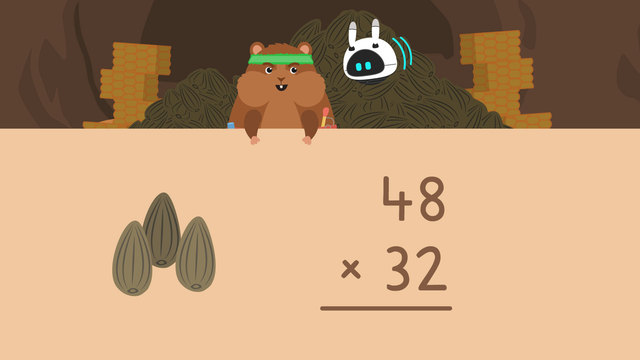# Multiplying Two-Digit Numbers by Two-Digit NumbersRate this video

Be the first to rate this video!

The authorTeam Digital
Multiplying Two-Digit Numbers by Two-Digit Numbers
CCSS.MATH.CONTENT.4.NBT.B.5

## Information about the videoMultiplying Two-Digit Numbers by Two-Digit Numbers

### In this Video on Multiplying 2-digit Numbers by 2-digit Numbers...

Mr. Squeaks and Imani are harvesting some food into containers for winter. However, they need help calculating how much of each food they have. We can help them by learning how to multiply by double digits.

#### Multiplying Two-Digit Numbers by Two-Digit Numbers

How do you do two digit multiplication? How do you multiply 2 digit numbers by 2 digit numbers without regrouping? Multiplying by two digit numbers can be done in a few easy steps:

• Multiply the one place in the bottom factor by both place values in the top factor, regrouping as needed.
• Add a placeholder, or zero
• Multiply the tens place in the bottom factor by both place values in the top factor, regrouping as needed

If you are still wondering how to do double digit multiplication, look at the example below.

#### Multiplying Two Digit by Two Digit Numbers Example

In this example, we will be multiplying by 2-digit numbers in order to find out how many sunflower seeds they have. Since there are forty-eight containers with thirty-two in each, we will find the product of forty-eight and thirty-two.

The first step is to multiply the ones place in both factors, so we multiply two times eight to get sixteen, regrouping the one to the tens place. Next multiply two times four to get eight, adding the one we regrouped to get nine.The second step is to add the placeholder, or zero.The third step is to multiply the tens place in the bottom factor by both place values in the top factor. We multiply three and eight to get twenty-four, and regroup the two to the tens place. Next multiply three times four to get twelve, adding the two we regrouped to get fourteen.The last step is to add the partial products ninety-six and one thousand four hundred forty to get the final product. Forty-eight times thirty-two is one thousand five hundred thirty-six.#### Multiplying Two Digit Numbers by Two Digit Numbers Summary

Remember, if someone asks you “how did you multiply 2 digit numbers by 2 digit numbers?” you can tell them to follow these easy steps:

• Multiply the one place in the bottom factor by both place values in the top factor, regrouping as needed.
• Add a placeholder, or zero
• Multiply the tens place in the bottom factor by both place values in the top factor, regrouping as needed